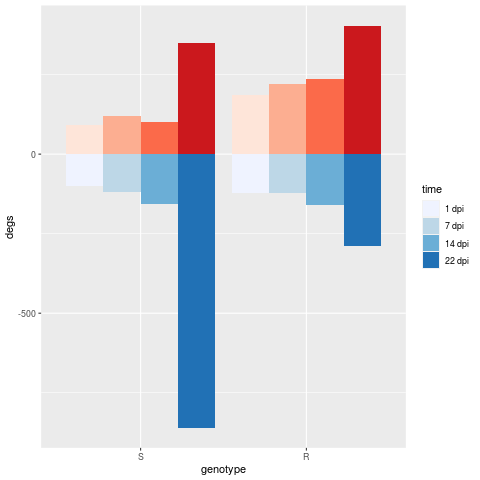Question: Add Both Legends to a Plot
0
pthom0100 wrote:I have a plot that I have made counting the number of genes over a period of time in two samples. I would like to plot both sets of numbers in a waterfall chart. I am able to get the chart but I have difficulty getting both legends to show up. I have the following tables:

``````deg.up
genotype   time degs
1         S  1 dpi   91
2         S  7 dpi  121
3         S 14 dpi  102
4         S 22 dpi  349
9         R  1 dpi  186
10        R  7 dpi  220
11        R 14 dpi  236
12        R 22 dpi  404

deg.dn
genotype   time degs
5         S  1 dpi  -99
6         S  7 dpi -120
7         S 14 dpi -157
8         S 22 dpi -860
13        R  1 dpi -121
14        R  7 dpi -122
15        R 14 dpi -161
16        R 22 dpi -288
``````

I am using the following code to make the plot:

``````deg.dn\$degs=deg.dn\$degs*-1
deg.up\$time=as.character(deg.up\$time)
deg.dn\$time=as.character(deg.dn\$time)
deg.up\$time = factor(deg.up\$time, levels=unique(deg.up\$time))
deg.dn\$time = factor(deg.dn\$time, levels=unique(deg.dn\$time))
deg.up\$genotype=as.character(deg.up\$genotype)
deg.dn\$genotype=as.character(deg.dn\$genotype)
deg.dn\$genotype = factor(deg.dn\$genotype, levels=unique(deg.dn\$genotype))
deg.up\$genotype = factor(deg.up\$genotype, levels=unique(deg.up\$genotype))
breaksup = levels(deg.up\$time)
breaksdn = levels(deg.dn\$time)
brewup = c("#FEE5D9", "#FCAE91", "#FB6A4A", "#CB181D")
brewdn = c("#EFF3FF", "#BDD7E7", "#6BAED6", "#2171B5")

ggplot() +
geom_bar(data = deg.up, aes(x=genotype, y=degs, fill=time), stat="identity", position="dodge") +
scale_fill_manual(values=brewup, breaks=breaksup) +
new_scale_fill() +
geom_bar(data = deg.dn, aes(x=genotype, y=degs, fill=time), stat="identity", position="dodge") +
scale_fill_manual(values=brewdn, breaks=breaksdn)
``````

Would anybody be able to help me get both legends next to each other in the final plot? Thank you.!

R • 153 views
modified 4 months ago by antonioggsousa1.9k • written 4 months ago by pthom0100
0
antonioggsousa1.9k wrote:

Hi,

If you want to keep your code as it is just add the parameter `name` to `scale_fill_manual()`, like this:

``````ggplot() +
geom_bar(data = deg.up, aes(x=genotype, y=degs, fill=time), stat="identity", position="dodge") +
scale_fill_manual(values=brewup, breaks=breaksup, name = "up") +
ggnewscale::new_scale_fill() +
geom_bar(data = deg.dn, aes(x=genotype, y=degs, fill=time), stat="identity", position="dodge",
legend) +
scale_fill_manual(values=brewdn, breaks=breaksdn, name = "down")
``````